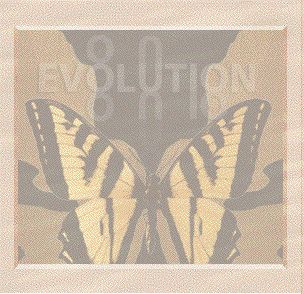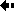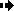## Genotype frequencyGenotype frequency and gene frequency are closely related variables, and both are easy to measure. The simplest case is one genetic locus with two alleles (A and a) and three genotypes (AA, Aa, and aa). Each individual has a genotype made up of two genes at the locus and a population can be symbolized like this:

Aa AA aa aa AA Aa AA Aa

This is an imaginary population with only eight individuals. To find the genotype frequencies we simply count the numbers of individual with each genotype. Thus:

frequency of AA = 3/8 = 0.375
frequency of Aa = 3/8 = 0.375
frequency of aa = 2/8 = 0.25

In general we can symbolize genotype frequencies algebraically, as follows.

 genotypeAA frequency Aa aa P Q R

P, Q, and R are expressed as percentages or proportions, so in our population, P = 0.375, Q = 0.375, R = 0.25 (they have to add up to one, or to 100%). They are measured simply by observing and counting the numbers of each type of organism in the population, and dividing by the total number of organisms in the population (the population size).Previous Next